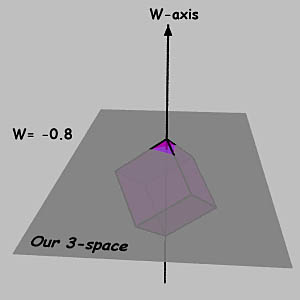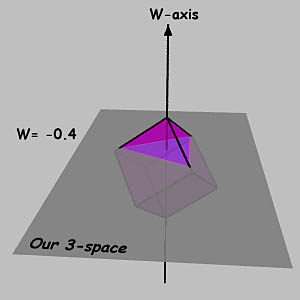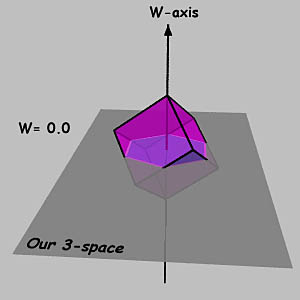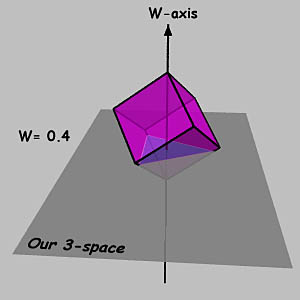## A 3-Dimensional Analogy to HyperSpace Polytope Slicing

This web page was created as an aid to understanding the HyperSlice Java applet, which displays 3-dimensional slices of 4-dimensional convex polytopes. It is equally relevant to the newer HyperStar Java applet.

In the images (below), a polytope (a cube) is dragged vertex-first across a lower-dimensional space (the gray plane). The (flat) inhabitants of the lower-dimensional space see a continuously-changing polytope in their space (a blue polygon which represents the intersection of the cube with the gray plane).

The W value is the distance by which the higher-dimensional polytope is shifted relative to the lower-dimensional space. In the HyperSlice applet, this is controlled by the W-Slider control. In the HyperStar applet, this is controled by the Section Slider control.

### The Images

 W= -0.8 Slice = Small TriangleW= -0.4 Slice = Large TriangleW= 0.0 Slice = HexagonW= 0.4 Slice = Large TriangleW= 0.8 Slice = Small TriangleEmail: Mark Newbold